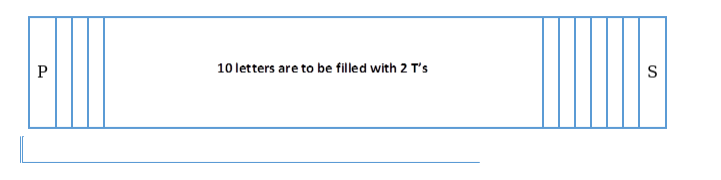# In how many ways can the letters of the word ‘PERMUTATIONS’

Question:

In how many ways can the letters of the word ‘PERMUTATIONS’ be arranged if each word starts with P and ends with S?

Solution:

Given: We have 12 letters

To Find: Number of words formed with Letter of the word ‘PERMUTATIONS.’

The formula used: The number of permutations of $n$ objects, where $p_{1}$ objects are of one kind, $\mathrm{p}_{2}$ are of the second kind, ..., $\mathrm{p}_{\mathrm{k}}$ is of $\mathrm{a} \mathrm{k}^{\text {th }}$ kind and the rest if any, are of a

different kind is $=\frac{n !}{p_{1} ! p_{2} ! \ldots \ldots \ldots \ldots p_{k} !}$

In the word 'PERMUTATIONS' we have $2 \mathrm{~T}$ 's.

We have to start the word with $\mathrm{P}$ and end it with $\mathrm{S}$, hence the first and last position is occupied with $P$ and $S$ respectively.

As two positions are occupied the remaining 10 positions are to be filled with 10 letters in which we have $2 \mathrm{~T}^{\prime} \mathrm{s}$.

NOTE:- Unless specified, assume that repetition is not allowed.

Let us represent the arrangementHence,

The first place is occupied by $P=1$ way

The last place $\left(12^{\text {th }}\right)$ is occupied by $S=1$ way

For the remaining 10 places:

Using the above formula

Where,

n=10

$\mathrm{p}_{1}=2$

$\Rightarrow \frac{10 !}{2 !}=1814400$

Total number of ways are $1 \times 1814400 \times 1=1814400$ ways.

In 1814400 ways the letters of the word 'PERMUTATIONS' can be arranged if each word starts with $P$ and ends with $S$.